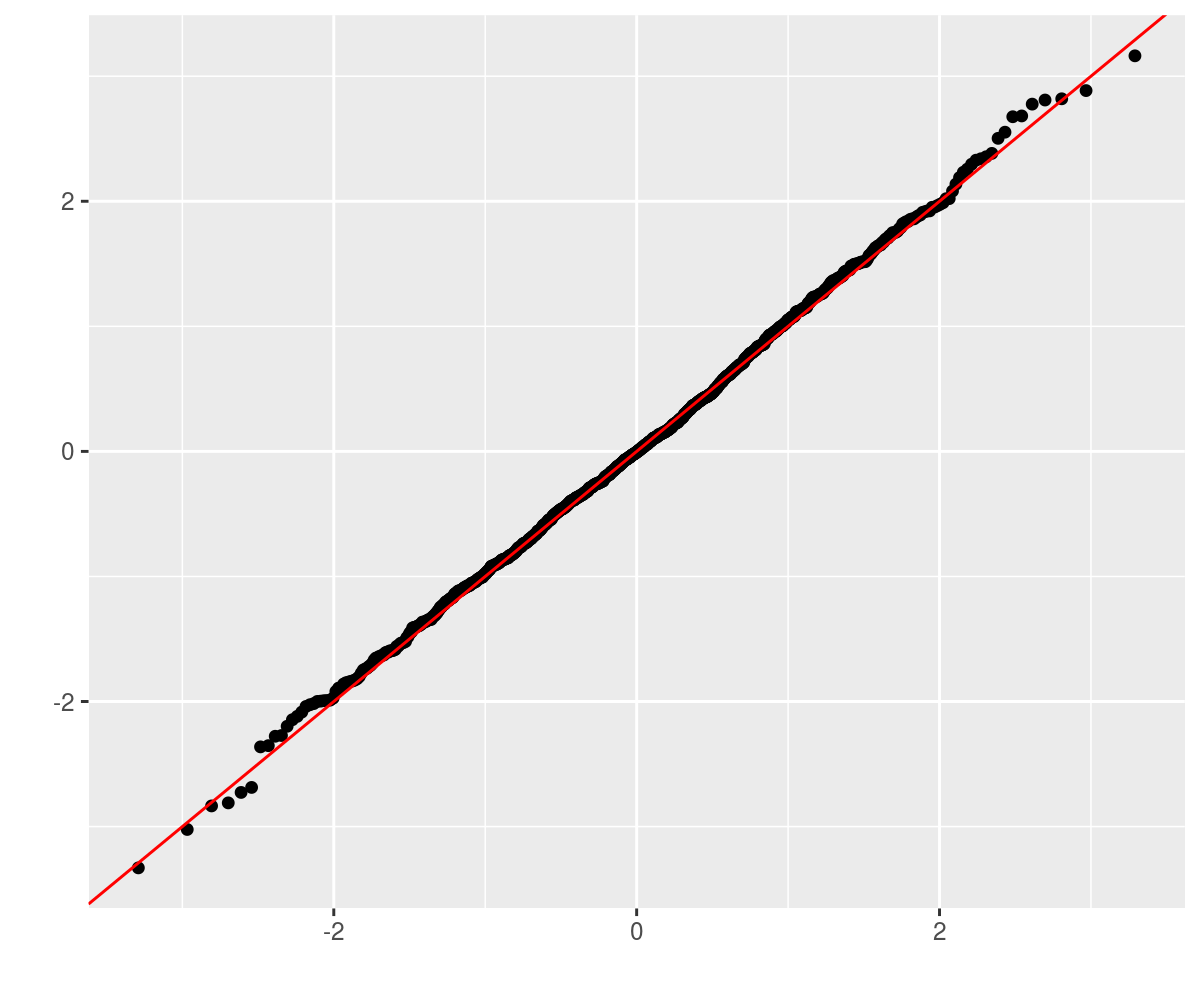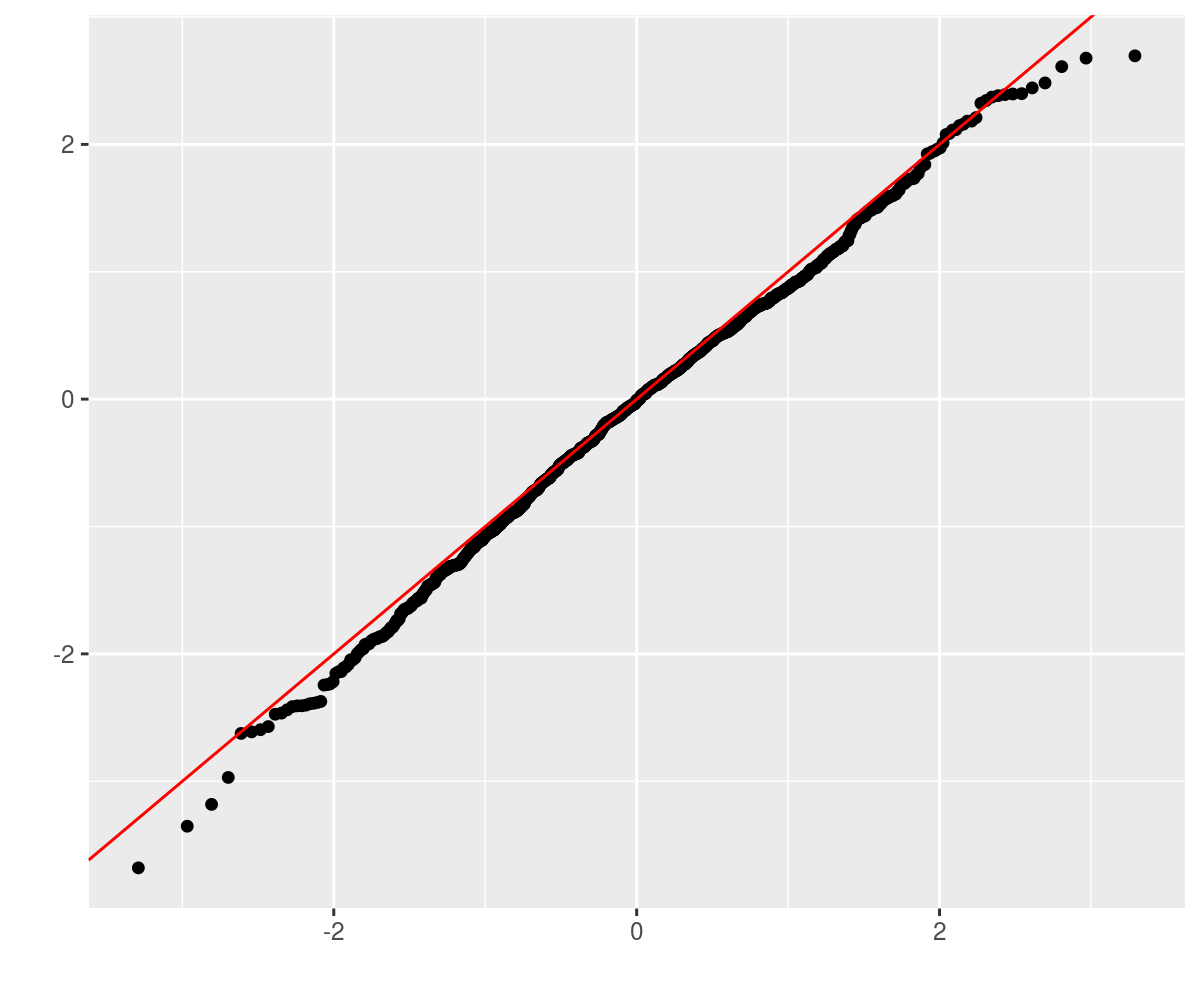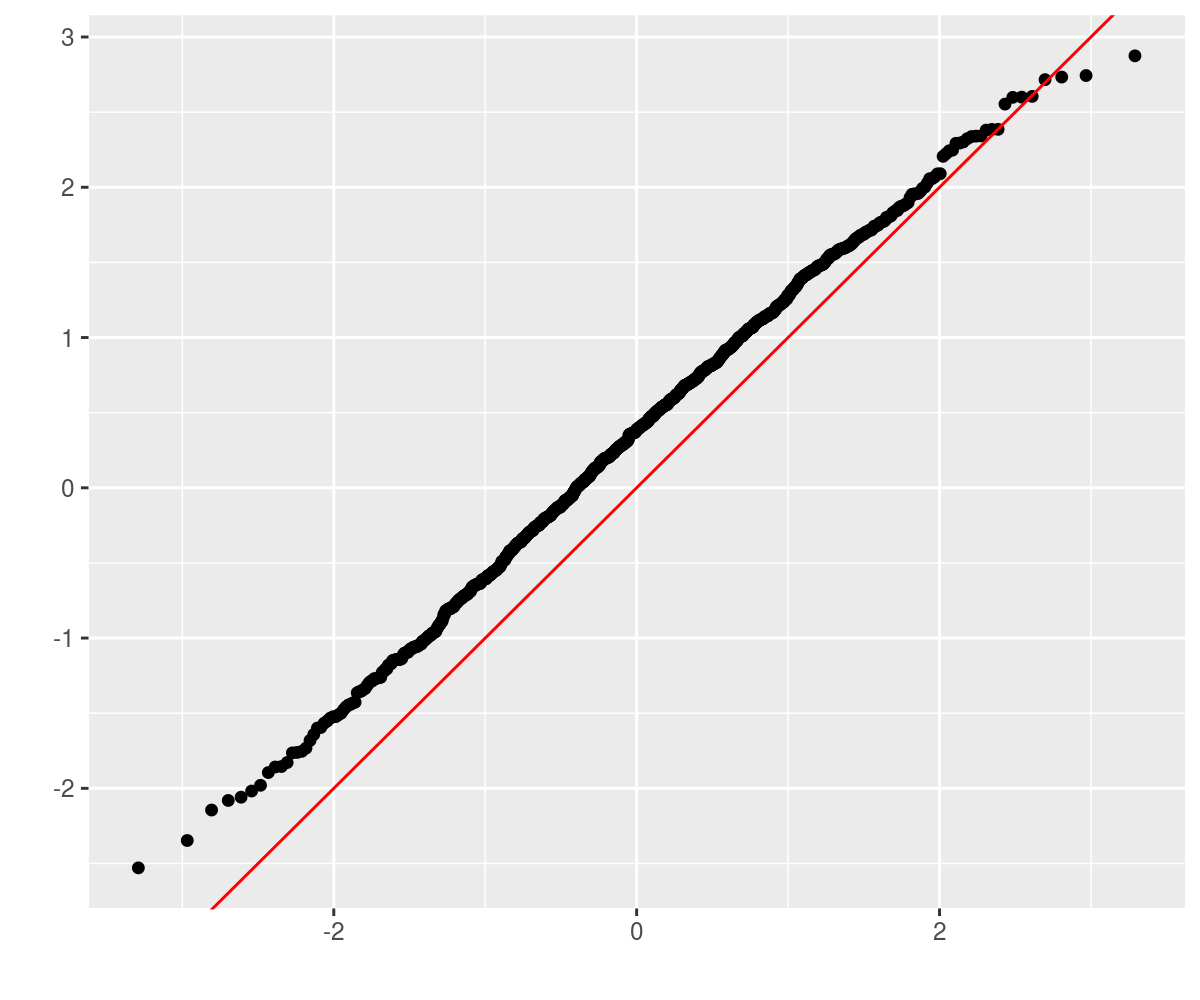# Automatic Differentiation of Multivariate Operations

An object that supports automatic differentiation of matrix- and multidimensional-valued functions with respect to multidimensional independent variables. Automatic differentiation is via 'forward accumulation'.

'madness' is a 'Multivariate Automatic Differentiation' package for R. It allows one to compute and track the derivative of multivariate to multivariate functions applied to concrete data via forward differentiation and the chain rule. The obvious use cases are for computing approximate standard errors via the Delta method, possibly for optimization of objective functions over vectors of parameters, and party tricks.

-- Steven E. Pav, [email protected]

## Installation

This package can be installed from CRAN, via drat, or from github:

# Basic Usage

You can store an initial value in a `madness` object, its derivative with respect to the independent variable, along with the 'name' of the dependent and independent variables, and an optional variance-covariance matrix of the independent variable. These are mostly filled in with sane defaults (the derivative defaults to the identity matrix, and so on):

``````## class: madness
##         d y
##  calc: -----
##         d x
##   val: -1.2 -0.56 ...
##  dvdx: 1 0 0 0 0 0 0 0 0 0 0 0 0 0 0 0 ...
##  varx:  ...
``````

You can then perform operations on objects and the derivative will be propagated forward via the chain rule:

``````## class: madness
##         d cbind((t((numeric * y)) %*% (numeric * y)), numeric)
##  calc: --------------------------------------------------------
##                                   d x
##   val: 37 5.9 1 0 ...
##  dvdx: -9.7 2.2 8.7 -19 3.4 4 -4.6 -4.4 0 0 0 0 0 0 0 0 ...
##  varx:  ...
``````
``````## class: madness
##         d colSums(cbind((t((numeric * y)) %*% (numeric * y)), numeric))
##  calc: -----------------------------------------------------------------
##                                       d x
##   val: 43 ...
##  dvdx: -12 -1.3 6.8 -23 0.33 4.3 -0.76 -4.8 -4.8 1.1 4.3 -9.4 1.7 2 -2.3 -2.2 ...
##  varx:  ...
``````
``````## class: madness
##         d norm(log(abs((numeric + (cbind((t((numeric * y)) %*% (numeric * y)), numeric) ^ numeric)))), 'f')
##  calc: -----------------------------------------------------------------------------------------------------
##                                                         d x
##   val: 10 ...
##  dvdx: -0.87 -0.72 -0.11 -1.6 -0.58 0.21 0.7 -0.26 -1.4 -0.23 0.72 -2.7 -0.031 0.49 -0.0021 -0.56 ...
##  varx:  ...
``````

## Variance-Covariance

You can optionally attach the variance-covariance matrix of the 'X' variable to a `madness` object. Then the estimated variance-covariance of computed quantities can be retrieved via the `vcov` method:

``````##          [,1]     [,2]     [,3]
## [1,]  0.00096 -2.0e-05 -1.4e-03
## [2,] -0.00002  2.5e-04  1.2e-05
## [3,] -0.00145  1.2e-05  2.9e-03
``````
``````## class: madness
##         d sqrt((t(val) %*% val))
##  calc: --------------------------
##                   d val
##   val: 3.6 ...
##  dvdx: 0.0037 0.55 -0.83 ...
##  varx: 0.00096 -2e-05 -0.0014 ...
``````
``````##        [,1]
## [1,] 0.0021
``````# Markowitz portfolio

There are two utilities for easily computing `madness` objects representing the first two moments of an object. Here we use these to compute the Markowitz portfolio of some assets, along with the estimated variance-covariance of the same. Here I first download the monthly simple returns of the Fama French three factors. (Note that you cannot directly invest in these, except arguably 'the market'. The point of the example is to use realistic returns, not provide investing advice.)

Now compute the first two moments via `twomoments` and compute the Markowitz portfolio

``````##      [,1]
## [1,] 2.80
## [2,] 0.33
## [3,] 2.19
``````
``````##       [,1]  [,2]  [,3]
## [1,]  0.47 -0.18 -0.13
## [2,] -0.18  0.87 -0.14
## [3,] -0.13 -0.14  0.76
``````
``````##      [,1]
## [1,] 4.07
## [2,] 0.35
## [3,] 2.51
``````

## Does that really work?

I don't know. Let's perform a bunch of simulations to see if the Wald statistics are OK. We will create a population with 5 stocks where the true Markowitz portfolio is -2,-1,0,1,2. We will perform 1000 simulations of 1250 days of returns from that population, computing the Markowitz portfolio each time. Then take the difference between the estimated and true Markowitz portfolios, divided by the standard errors.

This should be approximately normal, so we Q-Q plot against normality. LGTM.# Trace of the covariance matrix

Consider the case of an 8-vector drawn from some population. One observes some fixed number of independent observations of the vector, computes the sample covariance matrix, then computes its trace. Using a `madness` object, one can automagically estimate the standard error via the delta method. The sample calculation looks as follows:# Maximum eigenvalue of the covariance matrix

Consider the case of a 10-vector drawn from a population with covariance matrix whose largest eigenvalue is, say, 17.
One observes some fixed number of independent observations of the 10-vector, and computes the sample covariance matrix, then computes the maximum eigenvalue. Using a `madness` object, one can automagically estimate the standard error via the delta method. The sample calculation looks as follows:

``````## class: madness
##         d maxeig(Sigma)
##  calc: -----------------
##               d X
##   val: 18 ...
##  dvdx: 0 1.6 0.0068 -0.16 0.05 -0.0065 0.051 -0.0024 0.032 -0.035 -0.049 0.99 0.0086 -0.2 0.063 -0.0081 0.064 -0.003 0.04 -0.044 -0.062 1.9e-05 -0.00087 0.00028 -3.5e-05 0.00028 -1.3e-05 0.00018 -0.00019 -0.00027 0.01 -0.0064 0.00082 -0.0065 0.00031 -0.0041 0.0045 0.0063 0.001 -0.00026 0.0021 -9.6e-05 0.0013 -0.0014 -0.002 1.7e-05 -0.00026 1.2e-05 -0.00017 0.00018 0.00025 0.001 -9.8e-05 0.0013 -0.0014 -0.002 2.3e-06 -6.1e-05 6.7e-05 9.4e-05 0.00041 -9e-04 -0.0013 0.00049 0.0014 0.00097 ...
##  varx: 2.4e-34 9.4e-21 4.2e-20 1.8e-20 1.5e-20 -1.1e-20 -1.1e-20 2.6e-20 -7.5e-21 -4.2e-20 -1.6e-20 2e-19 -6e-20 -3e-20 -2e-20 2.3e-20 2.1e-20 -3.9e-20 1.6e-20 6.4e-20 1.5e-20 5.8e-20 -2.5e-20 -4.4e-20 6.4e-20 5.4e-20 -8.8e-20 3.2e-20 1.4e-19 3.5e-20 1.3e-19 -8.1e-21 2.3e-20 6.6e-21 -1.7e-20 1.5e-20 3.8e-20 6.7e-21 1.6e-19 2.3e-20 1.9e-20 -2.8e-20 7.3e-21 5.9e-20 1e-20 6e-21 -2.1e-20 4.3e-20 -1.5e-20 -7.6e-20 -2.3e-20 1.4e-19 2.6e-20 -1.1e-20 -5e-20 -1.7e-20 4.3e-20 1.8e-20 1e-19 2.8e-20 8.9e-20 -3.9e-20 -1.1e-20 -4.4e-20 -4.4e-20 1.4e-20 ...
``````
``````##      [,1]
## [1,] 0.53
``````

Now perform some simulations to see if these are accurate:Why the bias? There are a number of possibilities:

1. Broken example. Am I really checking what I intended?
2. Broken derivative code. Easy to check.
3. Failure to take symmetry into account.
4. Sample size not large enough. (ha ha.)
5. Derivative equal or near zero, requiring second term expansion in delta method.

# Enough already, bring me some Scotch!

An example is in order. Consider the tasting data compiled by Nessie on 86 Scotch whiskies. The data are availble online and look like so:

RowID Distillery Body Sweetness Smoky Medicinal Tobacco Honey Spicy Winey Nutty Malty Fruity Floral Postcode Latitude Longitude
1 Aberfeldy 2 2 2 0 0 2 1 2 2 2 2 2 PH15 2EB 286580 749680
2 Aberlour 3 3 1 0 0 4 3 2 2 3 3 2 AB38 9PJ 326340 842570
3 AnCnoc 1 3 2 0 0 2 0 0 2 2 3 2 AB5 5LI 352960 839320
4 Ardbeg 4 1 4 4 0 0 2 0 1 2 1 0 PA42 7EB 141560 646220
5 Ardmore 2 2 2 0 0 1 1 1 2 3 1 1 AB54 4NH 355350 829140
6 ArranIsleOf 2 3 1 1 0 1 1 1 0 1 1 2 KA27 8HJ 194050 649950

A bizarre question one could ask of this data are whether the taste characteristics are related to the geographic coordinates of the distilleries? One way to pose this is to perform a linear regression of the taste values on the geographic coordinates. This is a many-to-many regression. The Multivariate General Linear Hypothesis is a general hypothesis about the regression coefficients in this case. The 'usual' application is the omnibus test of whether all regression coefficients are zero. The MGLH is classically approached by four different tests, which typically give the same answer.

First, we grab the geographic and taste data, prepend a one to the vector, take an outer product and compute the mean and covariance. The MGLH statistics can be posed in terms of the eigenvalues of a certain matrix. Here these are statistics are computed so as to equal zero under the null hypothesis of all zero linear regression coefficient from geography to taste. We get the approximate standard errors from the delta method, and compute Wald statistics.

type stat Wald.stat
HLT 66.6 5.0
PBT 1.7 15.6
LRT 5.2 16.8
RLR 76.0 4.8

These all cast doubt on the hypothesis of 'no connection between geography and taste', although I am accustomed to seeing Wald statistics being nearly equivalent. This is still beta code. We also have the estimated standard error covariance of the vector of MGLH statistics, turned into a correlation matrix here. The four test methods have positively correlated standard errors, meaning we should not be more confident if all four suggest rejecting the null.

``````##      [,1] [,2] [,3] [,4]
## [1,] 1.00 0.15 0.75 0.98
## [2,] 0.15 1.00 0.75 0.11
## [3,] 0.75 0.75 1.00 0.71
## [4,] 0.98 0.11 0.71 1.00
``````

# Correctness

The following are cribbed from the unit tests (of which there are never enough). First we define the function which computes approximate derivatives numerically, then test it on some functions:

## Symmetry

Some functions implicitly break symmetry, which could cause the differentiation process to fail. For example, the 'chol' function is to be applied to a symmetric matrix, but only looks at the upper triangular part, ignoring the lower triangular part. This is demonstrated below. For the moment, the only 'solution' is to enforce symmetry of the input. Eventually some native functionality around symmetry may be implemented.

The functions `twomoments` and `theta` respect the symmetry of the quantities being estimated.

# Warnings

This code is a proof of concept. The methods used to compute derivatives are not (yet) space-efficient or necessarily numerically stable. User assumes all risk.

Derivatives are stored as a matrix in 'numerator layout'. That is the independent and dependent variable are vectorized, then the derivative matrix has the same number of columns as elements in the dependent variable. Thus the derivative of a 3 by 2 by 5 y with respect to a 1 by 2 by 2 x is a 30 by 4 matrix.

# Reference manual

install.packages("madness")

0.2.7 by Steven E. Pav, a year ago

https://github.com/shabbychef/madness

Report a bug at https://github.com/shabbychef/madness/issues

Browse source code at https://github.com/cran/madness

Authors: Steven E. Pav [aut, cre]

Documentation:   PDF Manual

Task views:

LGPL-3 license

Imports Matrix, matrixcalc, expm, methods

Suggests testthat, dplyr, tidyr, lubridate, SharpeR, sandwich, formatR, knitr

Depended on by rjmcmc.

Suggested by nlmixr.

See at CRAN